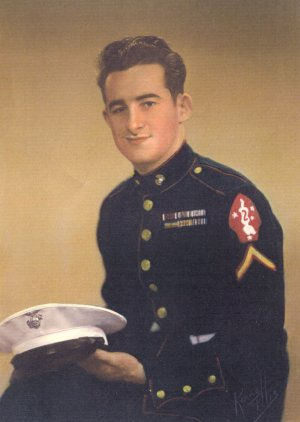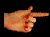ONE MAN'S VIEW: THE BOOKONE MAN'S VIEW is the personal story of Leonard Skinner's experiences in the Marine Corps during World War II. After enlisting at the age of 17 in January of 1942, Leonard spent four harrowing years moving from island to island in the Pacific Theatre. In this online book, One Man's View, he documents the hazardous life of an American Marine combat soldier during the war and reflects upon the experience of revisiting many of the battle sites half a century later.SITE CONTENTS PREFACE CONTENTS 1 INTRODUCTION 2 BOOT CAMP & BEYOND 3 GAVUTU & TANAMBOGO 4 GUADALCANAL 5 TULAGI 6 NEW ZEALAND 7 STATESIDE 8 HAWAII 9 SAIPAN 10 TINIAN 11 BACK TO SAIPAN 12 OKINAWA 13 AFTERMATH OF WAR 14 EPILOGUE APPENDIX ABOUT THE AUTHOR FIGURES: Fig. 1 (Chapter 1) Fig. 2 (Chapter 2) Fig. 3 (Chapter 2) Fig. 4 (Chapter 2) Fig. 5 (Chapter 2) Fig. 6 (Chapter 3) Fig. 7 (Chapter 3) Fig. 8 (Chapter 3) Fig. 9 (Chapter 3) Fig. 10 (Chapter 3) Fig. 11 (Chapter 3) Fig. 12 (Chapter 3) Fig. 13 (Chapter 3) Fig. 14 (Chapter 3) Fig. 15 (Chapter 3) Fig. 16 (Chapter 3) Fig. 17 (Chapter 3) Fig. 18 (Chapter 3) Fig. 19 (Chapter 3) Fig. 20 (Chapter 3) Fig. 21 (Chapter 3) Fig. 22 (Chapter 3) Fig. 23 (Chapter 4) Fig. 24 (Chapter 4) Fig. 25 (Chapter 4) Fig. 26 (Chapter 4) Fig. 27 (Chapter 4) Fig. 28 (Chapter 4) Fig. 29 (Chapter 4) Fig. 30 (Chapter 4) Fig. 31 (Chapter 5) Fig. 32 (Chapter 5) Fig. 33 (Chapter 5) Fig. 34 (Chapter 5) Fig. 35 (Chapter 6) Fig. 36 (Chapter 6) Fig. 37 (Chapter 6) Fig. 38 (Chapter 6) Fig. 39 (Chapter 6) Fig. 40 (Chapter 6) Fig. 41 (Chapter 6) Fig. 42 (Chapter 6) Fig. 43 (Chapter 7) Fig. 44 (Chapter 7) Fig. 45 (Chapter 8) Fig. 46 (Chapter 8) Fig. 47 (Chapter 8) Fig. 48 (Chapter 8) Fig. 49 (Chapter 8) Fig. 50 (Chapter 9) Fig. 51 (Chapter 9) Fig. 52 (Chapter 9) Fig. 53 (Chapter 9) Fig. 54 (Chapter 9) Fig. 55 (Chapter 9) Fig. 56 (Chapter 9) Fig. 57 (Chapter 10) Fig. 58 (Chapter 10) Fig. 59 (Chapter 10) Fig. 60 (Chapter 10) Fig. 61 (Chapter 10) Fig. 62 (Chapter 10) Fig. 63 (Chapter 10) Fig. 64 (Chapter 11) Fig. 65 (Chapter 11) Fig. 66 (Chapter 11) Fig. 67 (Chapter 11) Fig. 68 (Chapter 11) Fig. 69 (Chapter 11) Fig. 70 (Chapter 11) Fig. 71 (Chapter 11) Fig. 72 (Chapter 11) Fig. 73 (Chapter 12) Fig. 74 (Chapter 12) Fig. 75 (Chapter 12) Fig. 76 (Chapter 12) Fig. 77 (Chapter 12) Fig. 78 (Chapter 14) Fig. 79 (Chapter 14) This smartphone edition was last modified: 06/01/2019 Visit the Webmaster